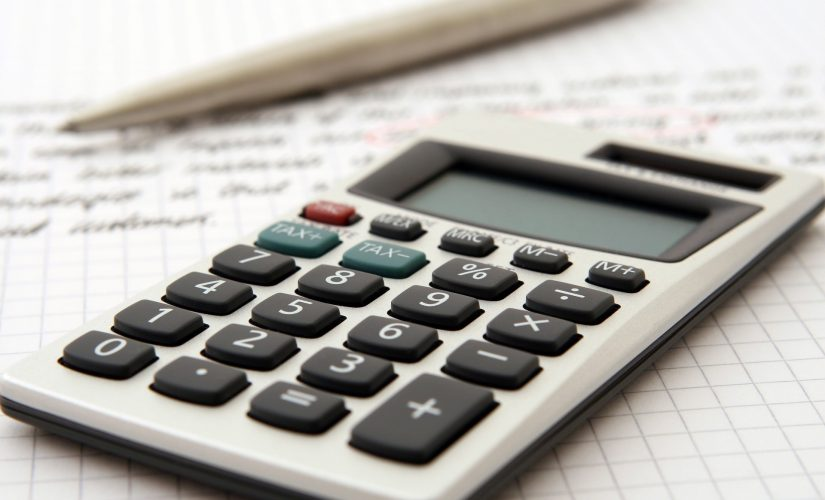# Learn How to Calculate Economic Order Quantity With a Simple Formula

The cost of inventory can weigh heavily and have an adverse impact on the profitability and performance of a business, which is why economic order quantity (EOQ) is a formula that is well worth getting to grips with as it helps you identify the optimum amount of stock that should be ordered each time in order optimize cost efficiency.

Here is a look at what economic order quantity is designed to achieve and why EOQ can be so fundamental to your efforts to achieve the best economies of scale and improve the company’s financial performance as a result of this efficiency.

### Ordering the right amount

As you will almost certainly appreciate if you are involved in running a business, EOQ is an important formula to have at your disposal as it helps you to identify the right amount of stock to order for maximum cost efficiency.

The problem you will often face is the fact that the cost of a unit can often be lower when you place a larger order, however, if you place an order that is too large it will have cost implications in terms of how much capital you have tied up in stock and it will also increase your overheads as you will have to pay for space needed to store the items.

### Crunching the numbers

The efficiency of EOQ is fundamentally underpinned by the accuracy of the data you input into the calculation.

There is a reasonably straightforward formula you can use to determine exactly how much of each product you should purchase each time so that you successfully maintain the most cost-efficient supply chain possible.

The basic formula involves a calculation for the anticipated level of demand, the order cost, and the holding cost.

It works on the basis that the cost per unit will decrease in line with the size of the order you place for an item but there is a tipping point when it becomes too costly to hold and carry that stock cost-effectively.

To arrive at the number the EOQ formula involves determining the demand level in units, the order cost, the holding cost, adjusted according to the incremental cost based on the proposed size of the order.

Once you have those numbers, simply multiply the demand figure by two before multiplying that number by the order cost. That number should then be divided by the holding cost figure.

You will find that a number of accounting packages offer an EOQ calculation option and that would also save you time as well as helping to eliminate an element of human error that could distort the figures.

The primary aim of using an EOQ formula is to help you identify the optimal number of units to order for maximum cost efficiency. It can also help you to manage cash flow better when you are more efficient with your ordering and inventory control.

EOQ is a simple formula that could transform the way you ordering your stock and it could also have a positive impact on your financial performance.